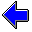Java Security Settings:
This web page employs Java, which requires specific security settings for correct operation.
If the applets on this page do not run correctly, consult the Virtual Chemistry Experiments FAQ
or the Physlet Physics web site for establishing the correct security settings.

# Spectrophotometry

## Determination of Analyte Concentration

One of the most common applications of spectrophotometry is to determine the concentration of an analyte in a solution. The experimental approach exploits Beer's Law, which predicts a linear relationship between the absorbance of the solution and the concentration of the analyte (assuming all other experimental parameters do not vary).

In practice, a series of standard solutions are prepared. A standard solution is a solution in which the analyte concentration is accurately known. The absorbances of the standard solutions are measured and used to prepare a calibration curve, which is a graph showing how the experimental observable (the absorbance in this case) varies with the concentration. For this experiment, the points on the calibration curve should yield a straight line (Beer's Law). The slope and intercept of that line provide a relationship between absorbance and concentration:

A = slope c + intercept

The unknown solution is then analyzed. The absorbance of the unknown solution, Au, is then used with the slope and intercept from the calibration curve to calculate the concentration of the unknown solution, cu.

 cu = Au - interceptslope

### Experiment

Objective:

• Determine the concentration of an unknown solution.

Approach:

• Measure the intensity of transmitted light for various standard solutions.
• For each standard solution, calculate the absorbance of the solution.
• Construct a calibration curve.
• Plot the line-of-best-fit through the experimental points.
• Measure the intensity of transmitted light for the unknown solution.
• Calculate the absorbance of the unknown solution.
• Use the calibration curve to determine the concentration of the unknown solution.

Run each simulation sufficiently long to detect at least 1000 photons. (Not all photons are shown on the screen.) Because the intensity for the blank is used to calculate all absorbances, it is especially important that the intensity for the blank be known accurately. If possible, wait until at least 4000 photons are detected for the blank.

 Concentration: blank 20.0 μmole L-1 standard solution 40.0 μmole L-1 standard solution 60.0 μmole L-1 standard solution 80.0 μmole L-1 standard solution unknown solution Number of Photons Detected: Elapsed Time: sec Intensity of Detected Light: photons/sec LightSource Cell Detector c = μmole L-1     A =     Plotted Points:                                                                                                                                                                                                           slope =           intercept =Beer's LawSpectrophotometry Home PageVirtual Chemistry Home Page

UnknownSolution.html version 2.1
© 2000-2014 David N. Blauch